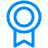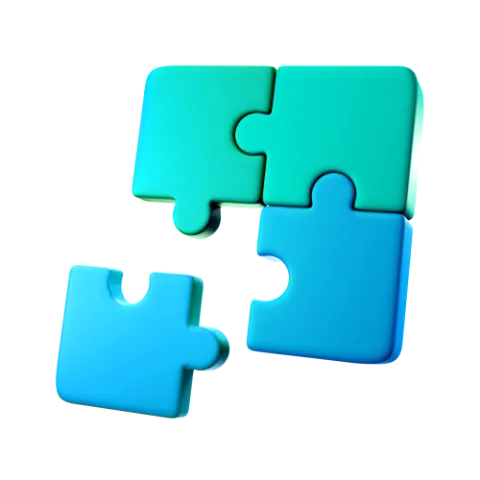Certificate

### Learn on Scaler Topics and get certified.# Convert Decimal to binary in C

Learn how to convert decimal to binary in C.

Updated - 1 Mar 20229 mins readPublished : 24 Nov 2021
Published : 24 Nov 2021Challenge Inside! : Find out where you stand! Try quiz, solve problems & win rewards!

## Overview

Given a binary number (a number that only consists of 0's and 1's) we can convert it into a decimal number (consists of numbers between 0 - 9) using loops, stacks, or bitwise operators.

This conversion is important because it is much more easier for a human to comprehend a decimal number compared to a binary number which is easier to operate on, by the machine.

## Scope

• In this article we will look at different types of number systems.
• We will also look at how to convert a number from decimal to binary form using 4 different techniques using loops, stacks, and bitwise operators.

## The Number System

The number system is basically used to represent the count of things. For example: How many rides are there in a circus? So more formally, Number System is a writing system for representing numbers using digits or symbols. In the number system, there are many ways to represent a number.

There are four main types of number systems:

• Binary System (base 2)
• Octal system (base 8)
• Decimal System (base 10)
• Hexadecimal system (base 16)

The base of a number system simply means a number of different digits a particular number system uses to represent numbers.

For example:

• base 2 means two digits (0,1) are used to represent numbers.
• base 10 means 10 digits (0,1,2,3,4,5,6,7,8,9) are used to represent numbers.

We can convert a number from one number system to any other number system.

In this article, we will learn how to convert a number from decimal to binary in C.

### What is Decimal Number?

A decimal number is a number represented in the decimal number system. This system uses base 10 to represent numbers i.e every digit in decimal number is one of the following numbers : 0,1,2,3,4,5,6,7,8,9.

eg: 123, 32, 45 etc.

### What is Binary Number?

Binary number is a number represented in the binary number system. This system uses base 2 to represent numbers i.e every digit in binary number is one of the following numbers: 0,1.

eg: 101, 111, 100 etc.

## Representation of Decimal and Binary Numbers

Binary number system uses only (0,1) to represent numbers and, Decimal number system uses (0,1,2,3,4,5,6,7,8,9) to represent numbers.

Below table shows decimal number and its corresponding representation in the binary number system

Decimal numberBinary Number
00000
10001
20010
30011
40100
50101
60110
70111
81000
91001

Now, let us understand why 9(in decimal) is equal to 1001(in binary).

9(in binary)1001
$2^3$$2^2$$2^1$$2^0$
place value1*80*40*21*1

To understand this we first have to understand the place value concept. Place value is simply the value associated with every digit in any number system and it is calculated from right to left in a number i.e from least significant bit to most significant bit.

In the above table consider the least significant digit(rightmost), which is 1 and the value associated with that place is $2^0$, therefore place value of the digit will be $2^0$ * 1. Similarly all place values are calculated and are added up to prove that 1001(base 2) = 8 + 0 + 0 + 1 = 9(base 10). That's how 9(in decimal) = 1001(in binary)

## Algorithm for Decimal to Binary Conversion in C

Step 1: Divide the number by 2 and find the remainder, then store the remainder in an array.

Step 2: Divide the number by 2.

Step 3: Repeat the above two steps until the number is greater than zero.

Step 4: Print the array in reverse order to get the binary representation of the number.

## Different Approaches to Convert Decimal Number to Binary Number in C

### Using for-loop

1. Run a for loop until a number is greater than 0.
2. Divide the number by 2 and find the remainder, then store the remainder in an array.
3. Divide the number by 2.
4. Repeat the above two steps until number is greater than zero.
5. Print the array in reverse order to get the binary representation of the number.
#include <stdio.h>

void decimalToBinary(int num) {
if (num == 0) {
printf("0");
return;
}

// Stores binary representation of number.
int binaryNum; // Assuming 32 bit integer.
int i=0;

for ( ;num > 0; ){
binaryNum[i++] = num % 2;
num /= 2;
}

// Printing array in reverse order.
for (int j = i-1; j >= 0; j--)
printf("%d", binaryNum[j]);
}

int main() {
int num = 9;
decimalToBinary(num);
return 0;
}


Output :

1001


Time Complexity: O(log n) because we keep on dividing the number by 2 in every step which overall takes log n steps.

Space Complexity: O(log n) because there are log n + 1 digits in the binary representation of a number.

### Using while loop

1. Run a while loop until a number is greater than 0.
2. Divide the number by 2 and find the remainder, then store the remainder in an array.
3. Divide the number by 2.
4. Repeat the above two steps until number is greater than zero.
5. Print the array in reverse order to get the binary representation of the number.
#include <stdio.h>

void decimalToBinary(int num) {

if (num == 0) {
printf("0");
return;
}

// Stores binary representation of number.
int binaryNum; // Assuming 32 bit integer.
int i=0;

while (num > 0) {
binaryNum[i++] = num % 2;
num /= 2;
}

// Printing array in reverse order.
for (int j = i-1; j >= 0; j--)
printf("%d", binaryNum[j]);
}

int main() {
int num = 9;
decimalToBinary(num);
return 0;
}


Output:

1001


Time Complexity: O(log n) because we keep on dividing the number by 2 in every step which overall takes log n steps.

Space Complexity: O(log n) because there are log n + 1 digits in the binary representation of a number.

### Using Stack

1. Run a while loop until a number is greater than 0.
2. Divide the number by 2 and find the remainder, then push the remainder into the top of the stack.
3. Divide the number by 2.
4. Repeat the above two steps until number is greater than zero.
5. Keep on printing the top element of the stack until stack is not empty to get the binary representation of the number.
#include <stdio.h>

// stores binary representation of number
int Stack;

// denotes stack is empty
int top = -1;

void push(int x) {
Stack[++top] = x;
}

void pop() {
top--;
}

void decimalToBinary(int num) {
if (num == 0) {
printf("0");
return;
}

while (num > 0) {

// Push into the stack.
push(num % 2);

num /= 2;
}

// Printing stack to get binary representation.
while (top != -1) {
printf("%d", Stack[top]);
pop();
}
}

int main() {
int num = 9;
decimalToBinary(num);
return 0;
}


Output:

1001


Time Complexity : O(log n) because we keep on dividing the number by 2 in every step which overall takes log n steps.

Space Complexity : O(log n) because there are log n + 1 digits in the binary representation of a number.

### Without Using Arrays

Idea here in this algorithm is not to use any extra space to store binary representation of the number. We do so by storing the binary representation as a decimal number.

Steps:

1. Run a while loop until a number is greater than 0 and maintain a varible power and binNumber initialised with 0.
2. Divide the number by 2 and find the remainder, then find the place value of the remainder which is rem * pow(10, power) and add it to binNumber.
3. Increment the power variable and divide the number by 2.
4. Repeat the above two steps until number is greater than zero.
5. Print binNumber to get the binary representation of the number.
#include <stdio.h>
#include <math.h>

void decimalToBinary(int num) {
long long int binNumber = 0;
int power = 0;

while (num > 0) {
int rem = num % 2;
long long int temp = pow(10, power);
binNumber += rem * temp;
power++;
num /= 2;
}

printf("%lld", binNumber);
}

int main() {
int num = 9;
decimalToBinary(num);
return 0;
}


Output :

1001


Time Complexity: O(log n) because we keep on dividing the number by 2 in every step which overall takes log n steps.

Space Complexity: O(1) because we are not using any extra space.

### Using Bitwise Operators

Idea here in this algorithm is to find binary representation of the number by checking every bit of the number. Since we know that any number is stored as binary in the computer system, so by checking every bit we will be able to find the required binary representation.

Steps:

1. Run a for loop from left to right in the number i.e from most significant bit to the least significant bit.
2. Check whether the bit is 0 or 1, we do so by taking 'bitwise and' of mask and num, if it is non-zero then current bit is 1 else current bit is 0.
3. Print the bit after every iteration to get binary representation of the number.
#include <stdio.h>

// this method will print all the 32 bits of a number
void decimalToBinary(int num) {
// assuming 32 bit integer
for (int i = 31; i >= 0; i--) {

// calculate bitmask to check whether
// ith bit of num is set or not
int mask = (1 << i);

// ith bit of num is set
if (num & mask)
printf("1");
// ith bit of num is not set
else
printf("0");
}
}

int main() {
int num = 5;
decimalToBinary(num);
return 0;
}


Output:

00000000000000000000000000000101


Time Complexity: O(log n) because there are log n + 1 digits in the binary representation of the number.

Space Complexity: O(1) because we are not using any extra space.

## Conclusion